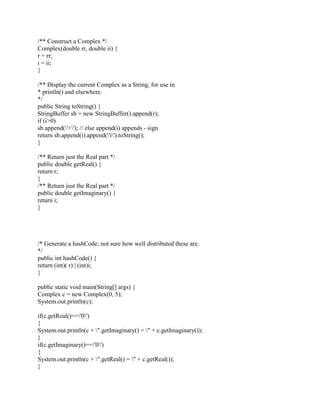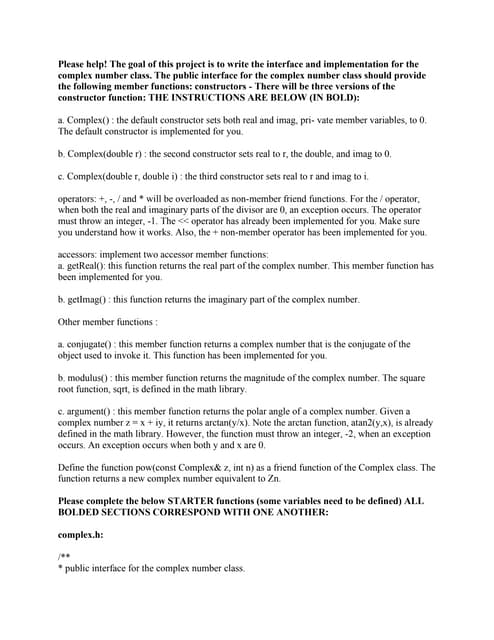AnúncioPróximos SlideSharesPlease help! The goal of this project is to write the interface and im.pdf
Carregando em ... 3
1 de 3
Anúncio

### (Modify)a- Determine why the abs() function call in the Complex classs.docx

1. (Modify)a. Determine why the abs() function call in the Complex classâ€™s showComplexValues() function is required for displaying the imaginaryPart data member but would be incorrect if applied to the realPart data member. b. Modify the Complex classâ€™s showComplexValues() function to display a complex number according to the following algorithm: If both the real and imaginary parts of a Complex number object are 0, the output should be 0 ElseIf only the imaginary part is 0, the output should display only the real part ElseIf only the real part is 0, the output should display only the imaginary part Else the output should include both a real and an imaginary part For example, the complex number 0 + 0i should be displayed as 0, the complex number 4.2 + 0i should be displayed as 4.2, the complex number 0 + 6.7i should be displayed as 6.7i, and the complex number 4.2 + 6.7i should be displayed as 4.2 + 6.7i. Solution /** A class to represent Complex Numbers. **/ public class Complex { /** The real part */ private double r; /** The imaginary part */ private double i;
2. /** Construct a Complex */ Complex(double rr, double ii) { r = rr; i = ii; } /** Display the current Complex as a String, for use in * println() and elsewhere. */ public String toString() { StringBuffer sb = new StringBuffer().append(r); if (i>0) sb.append('+'); // else append(i) appends - sign return sb.append(i).append('i').toString(); } /** Return just the Real part */ public double getReal() { return r; } /** Return just the Real part */ public double getImaginary() { return i; } /* Generate a hashCode; not sure how well distributed these are. */ public int hashCode() { return (int)( r) | (int)i; } public static void main(String[] args) { Complex c = new Complex(0, 5); System.out.println(c); if(c.getReal()=='0') { System.out.println(c + ".getImaginary() = " + c.getImaginary()); } if(c.getImaginary()=='0') { System.out.println(c + ".getReal() = " + c.getReal()); }
3. if((c.getReal()=='0')&(c.getImaginary()=='0')) { System.out.println("zero"); } else { System.out.println(c + ".getReal() = " + c.getReal()); System.out.println(c + ".getImaginary() = " + c.getImaginary()); } } }
Anúncio